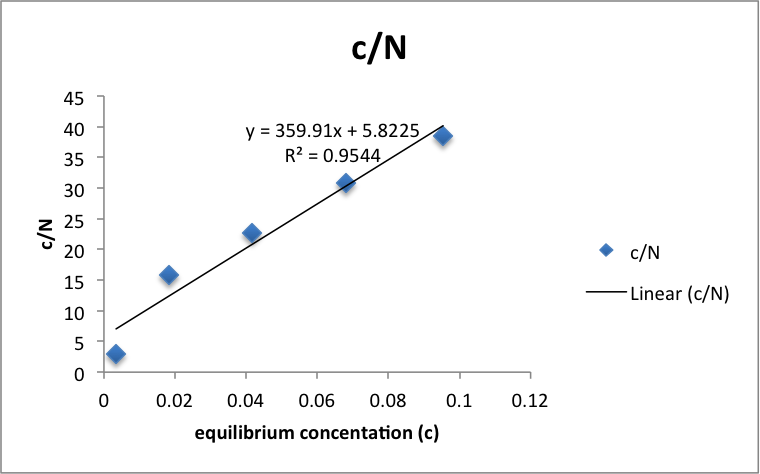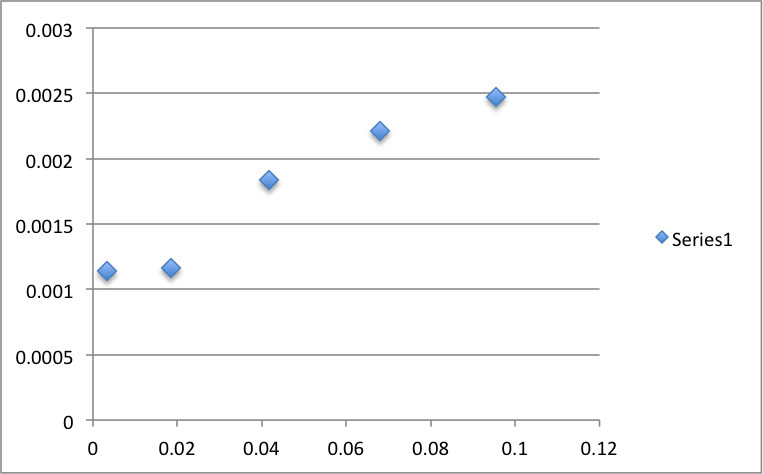# Adsorption of Aqueous Acetic Acid onto Activated Charcoal

Written by Javon

Results and Discussion

The results were obtained by the collaborators who performed the experiment.

Figure 1: c/N vs cTable 1: c/N vs c

 c c/N 0.00342342 3.00598832 0.01837836 15.831308 0.04160156 22.6250274 0.06804798 30.7722158 0.09537528 38.5146221 0.12260248 4.45704831

Using the equation c/N = c/Nm + 1/kNm, Nm (number of moles of acetic acid required to forma monolayer onto the charcoal) can be approximated as the inverse of the slope of the best fit line. The data and graph was organized and computed using Microsoft Excel. The Regression analysis tool calculated the error in the slope to be 0.05. Nm = .0028 ± 4×10-7 M/g.

Figure 2: Isotherm of c vs NTable 2: c vs N

 c N 0.00342342 0.00113887 0.01837836 0.00116089 0.04160156 0.00183874 0.06804798 0.00221134 0.09537528 0.00247634

Upon analysis of the isotherm, we see that the graph agrees with our experimental value. The graph plateaus around .0028 as calculated.

We can use this to calculate the area per gram of charcoal (specific area) using the equation

A = NmN0σ10-20

Where A is the specific area, N0 is Avogrado’s number, σ is a given area parameter unique to acetic acid equal to 21 angstroms.1 The specific area was calculated to be 354  ± .05 m2/g, 11.5% from the literature value of 400 m2/g.1

While pressure would not have much of an effect since this experiment deals with aqueous phase adsorption, temperature may greatly affect the amount of acetic acid absorbed onto the surface. Since the electrostatic bonds that bind the adsorbent to the solid in physisorption do not require as much energy to dissociate the chemical bonds do in chemisorption, less vigorous conditions such as changing temperature would be required to change in equilibrium concentration of adsorbent.

References

1. 1.     Milosavljevic, B.H. Lab Packet for CHEM 457: Experimental Physical Chemistry, Gravimetric Determination of the Gas Constant. University Press: University Park, 2009.
• Share